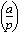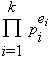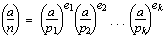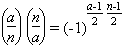# Jacobi symbol

Recall that the second (lower) entry in the Legendre symbol (a|q), also denoted, must be prime. Jacobi generalized the Legendre symbol to allow lower entries that are odd (but not necessarily prime) as follows: Let the factorization of n be. Then

•Notice that (a|1) = 1 for all integers a.

This new symbol (which looks just like the Legendre symbol) is called the Jacobi symbol. Warning: For the Jacobi symbol, (a|n)=1 does not necessarily mean that a is a quadratic residue of n. For example, (8|15) = 1, but 8 is not a quadratic residue of 15.

The Jacobi symbol has many properties that make its use the easiest way to evaluate a Legendre symbol. Suppose m and n are positive odd integers, and a and b are any integers. Then the Jacobi symbol satisfies the following:

• (a|n)=0 if gcd(a,n) > 1.
• (-1|n) = 1 if n ≡ 1 (mod 4), and (-1|n) = -1 if n ≡ 3 (mod 4);
• (a|n) (b|n) = (ab|n);
• (a|m) (a|n) = (a|mn);
• if ab (mod n), then (a|n) = (b|n).

For the prime 2 we have

• (2|n) = 1 if n ≡ 1 or 7 (mod 8), and
• (2|n) = -1 if n ≡ 3 or 5 (mod 8).

Finally, and perhaps most usefully, if gcd(a,n) =1, and a is odd and positive,

•In other words, (a|n)= (n|a), unless an ≡ 3 (mod 4), in which case (a|n) = -(n|a).

This gives the following algorithm for finding (a|n). Suppose n is odd and 0 < a < n:

``````Jacobi(a,n) {
j := 1
while (a not 0) do {
while (a even) do {
a := a/2
if (n ≡ 3 (mod 8) or n ≡ 5 (mod 8)) then j := -j
}
interchange(a,n)
if (a ≡ 3 (mod 4) and n ≡ 3 (mod 4)) then j := -j
a := a mod n
}
if (n = 1) then return (j) else return(0)
}
``````

Notice that this is just an adaptation of the classical Euclidean algorithm.